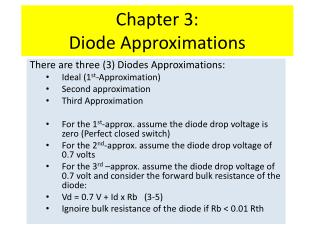DownloadDownload PresentationChapter 3: Diode Approximations

# Chapter 3: Diode Approximations

Télécharger la présentation## Chapter 3: Diode Approximations

- - - - - - - - - - - - - - - - - - - - - - - - - - - E N D - - - - - - - - - - - - - - - - - - - - - - - - - - -
##### Presentation Transcript

1. Chapter 3:Diode Approximations There are three (3) Diodes Approximations: Ideal (1st-Approximation) Second approximation Third Approximation For the 1st-approx. assume the diode drop voltage is zero (Perfect closed switch) For the 2nd-approx. assume the diode drop voltage of 0.7 volts For the 3rd –approx. assume the diode drop voltage of 0.7 volt and consider the forward bulk resistance of the diode: Vd = 0.7 V + Id x Rb (3-5) Ignoire bulk resistance of the diode if Rb < 0.01 Rth

2. Forward Bulk Resistance Calculate the Bulk Resistance (Rb) from the formula given below: Rb = (V2 – V1)/(I2 – I1) Forward bulk resistance (Rb) is very small less than 1 Ohms or 10 Ohms. The bulk resistance is the dynamic resistance while current pass through the diode, but static resistance that usually measured by meter is relatively small compare to the bulk resistance. The reverse resistance of the diode is very large greater than 1 M-ohms.

3. Example of 2nd-approximation

4. Thevenin’s Equvalect Circuit